Documentation

# creditexposures

Compute credit exposures from contract values

## Syntax

``````[exposures,exposurecpty] = creditexposures(values,counterparties)``````
``````[exposures,exposurecpty] = creditexposures(___,Name,Value)``````
``````[exposures,exposurecpty,collateral] = creditexposures(___,Name,Value)``````

## Description

example

``````[exposures,exposurecpty] = creditexposures(values,counterparties)``` computes the counterparty credit exposures from an array of mark-to-market OTC contract values. These exposures are used when calculating the CVA (credit value adjustment) for a portfolio. ```

example

``````[exposures,exposurecpty] = creditexposures(___,Name,Value)``` adds optional name-value arguments.```

example

``````[exposures,exposurecpty,collateral] = creditexposures(___,Name,Value)``` computes the counterparty credit exposures from an array of mark-to-market OTC contract values using optional name-value pair arguments for `CollateralTable` and `Dates`, the `collateral` output is returned for the simulated collateral amounts available to counterparties at each simulation date and over each scenario.```

## Examples

collapse all

After computing the mark-to-market contract values for a portfolio of swaps over many scenarios, compute the credit exposure for a particular counterparty. View the contract values and credit exposure over time. First, load data (`ccr.mat`) containing the mark-to-market contract values for a portfolio of swaps over many scenarios.

```load ccr.mat % Look at one counterparty. cpID = 4; cpValues = squeeze(sum(values(:,swaps.Counterparty == cpID,:),2)); subplot(2,1,1) plot(simulationDates,cpValues); title(sprintf('Mark-to-Market Contract Values for Counterparty: %d',cpID)); datetick('x','mmmyy') ylabel('Portfolio Value (\$)') % Compute the exposure by counterparty. [exposures, expcpty] = creditexposures(values,swaps.Counterparty,... 'NettingID',swaps.NettingID); % View the credit exposure over time for the counterparty. subplot(2,1,2) cpIdx = find(expcpty == cpID); plot(simulationDates,squeeze(exposures(:,cpIdx,:))); title(sprintf('Exposure for counterparty: %d',cpIdx)); datetick('x','mmmyy') ylabel('Exposure (\$)') xlabel('Simulation Dates')```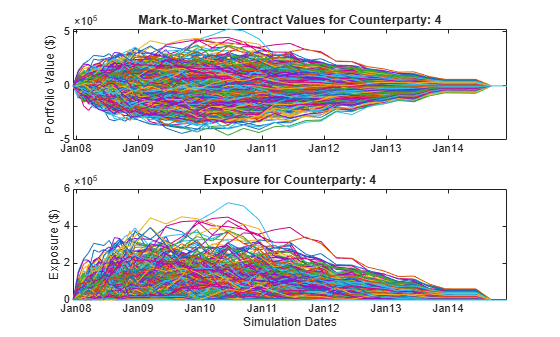Load the data (`ccr.mat`) containing the mark-to-market contract values for a portfolio of swaps over many scenarios.

`load ccr.mat`

Look at one counterparty.

```cpID = 4; cpIdx = swaps.Counterparty == cpID; cpValues = values(:,cpIdx,:); plot(simulationDates,squeeze(sum(cpValues,2))); grid on; title(sprintf('Potential Mark-to-Market Portfolio Values for Counterparty: %d',cpID)); datetick('x','mmmyy') ylabel('Portfolio Value (\$)')```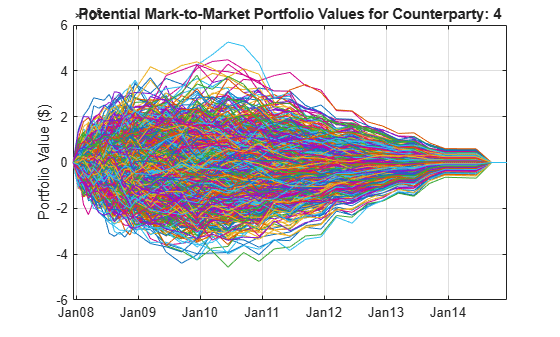Compute the exposures.

```netting = swaps.NettingID(cpIdx); exposures = creditexposures(cpValues,cpID,'NettingID',netting);```

View the credit exposure over time for the counterparty.

```figure; plot(simulationDates,squeeze(exposures)); grid on title(sprintf('Exposure for counterparty: %d',cpID)); datetick('x','mmmyy') ylabel('Exposure (\$)') xlabel('Simulation Dates')```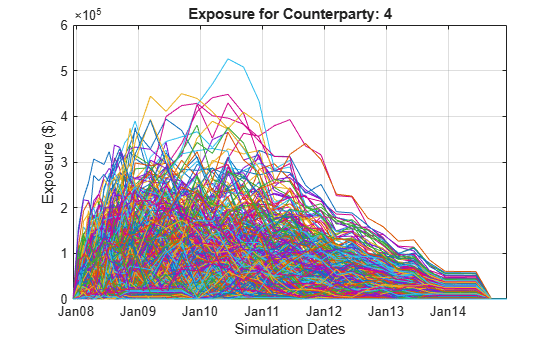Compute the credit exposure profiles.

`profilesBefore = exposureprofiles(simulationDates,exposures)`
```profilesBefore = struct with fields: Dates: [37x1 double] EE: [37x1 double] PFE: [37x1 double] MPFE: 2.1580e+05 EffEE: [37x1 double] EPE: 2.8602e+04 EffEPE: 4.9579e+04 ```

Consider a new trade with a counterparty. For this example, take another trade from the original swap portfolio and "copy" it for a new counterparty. This example is only for illustrative purposes.

```newTradeIdx = 3; newTradeValues = values(:,newTradeIdx,:); % Append a new trade to your existing portfolio. cpValues = [cpValues newTradeValues]; netting = [netting; cpID]; exposures = creditexposures(cpValues,cpID,'NettingID',netting);```

Compute the new credit exposure profiles.

`profilesAfter = exposureprofiles(simulationDates,exposures)`
```profilesAfter = struct with fields: Dates: [37x1 double] EE: [37x1 double] PFE: [37x1 double] MPFE: 2.4689e+05 EffEE: [37x1 double] EPE: 3.1609e+04 EffEPE: 5.6178e+04 ```

Visualize the expected exposures and the new trade's incremental exposure. The incremental exposure is used to compute the incremental credit value adjustment (CVA) charge.

```figure; subplot(2,1,1) plot(simulationDates,profilesBefore.EE,... simulationDates,profilesAfter.EE); grid on; legend({'EE before','EE with trade'}) datetick('x','mmmyy','keeplimits') title('Expected Exposure before and after new trade'); ylabel('Exposure (\$)') subplot(2,1,2) incrementalEE = profilesAfter.EE - profilesBefore.EE; plot(simulationDates,incrementalEE); grid on; legend('incremental EE') datetick('x','mmmyy','keeplimits') ylabel('Exposure (\$)') xlabel('Simulation Dates')```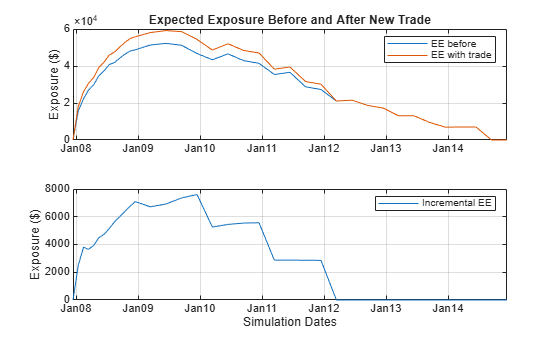Load the data (`ccr.mat`) containing the mark-to-market contract values for a portfolio of swaps over many scenarios.

`load ccr.mat`

Only look at a single counterparty for this example.

```cpID = 4; cpIdx = swaps.Counterparty == cpID; cpValues = values(:,cpIdx,:);```

Compute the uncollateralized exposures.

```exposures = creditexposures(cpValues,swaps.Counterparty(cpIdx),... 'NettingID',swaps.NettingID(cpIdx));```

View the credit exposure over time for the counterparty.

```plot(simulationDates,squeeze(exposures)); expYLim = get(gca,'YLim'); title(sprintf('Exposures for Counterparty: %d',cpID)); datetick('x','mmmyy') ylabel('Exposure (\$)') xlabel('Simulation Dates')```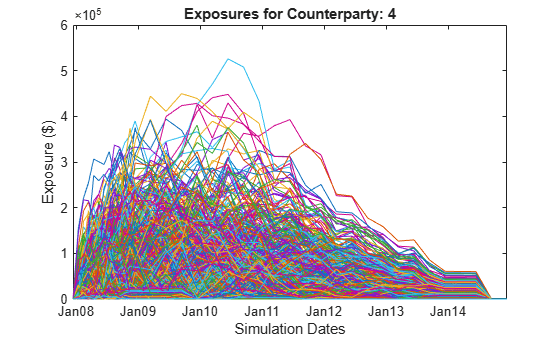Now add a collateral agreement for the counterparty. The `'CollateralTable'` parameter is a MATLAB® table. You can create tables from spreadsheets or other data sources, in addition to building them inline as seen here. For more information, see `table`.

```collateralVariables = {'Counterparty';'PeriodOfRisk';'Threshold';'MinimumTransfer'}; periodOfRisk = 14; threshold = 100000; minTransfer = 10000; collateralTable = table(cpID,periodOfRisk,threshold,minTransfer,... 'VariableNames',collateralVariables)```
```collateralTable=1×4 table Counterparty PeriodOfRisk Threshold MinimumTransfer ____________ ____________ _________ _______________ 4 14 1e+05 10000 ```

Compute the collateralized exposures.

```[collatExp, collatcpty, collateral] = creditexposures(cpValues,... swaps.Counterparty(cpIdx),'NettingID',swaps.NettingID(cpIdx),... 'CollateralTable',collateralTable,'Dates',simulationDates);```

Plot the collateral levels and collateralized exposures.

```figure; subplot(2,1,1) plot(simulationDates,squeeze(collateral)); set(gca,'YLim',expYLim); title(sprintf('Collateral for counterparty: %d',cpID)); datetick('x','mmmyy') ylabel('Collateral (\$)') xlabel('Simulation Dates') subplot(2,1,2) plot(simulationDates,squeeze(collatExp)); set(gca,'YLim',expYLim); title(sprintf('Collateralized Exposure for Counterparty: %d',cpID)); datetick('x','mmmyy') ylabel('Exposure (\$)') xlabel('Simulation Dates');```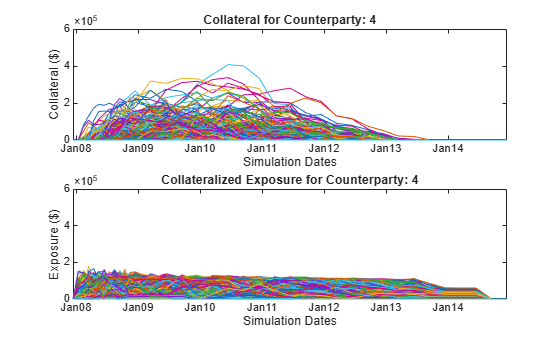## Input Arguments

collapse all

3-D array of simulated mark-to-market values of a portfolio of contracts simulated over a series of simulation dates and across many scenarios, specified as a `NumDates`-by-`NumContracts`-by-`NumScenarios` “cube” of contract values. Each row represents a different simulation date, each column a different contract, and each “page” is a different scenario from a Monte-Carlo simulation.

Data Types: `double`

Counterparties corresponding to each contract in `values`, specified as a `NumContracts`-element vector of counterparties. Counterparties can be a vector of numeric IDs or a cell array of counterparty names. By default, each counterparty is assumed to have one netting set that covers all of its contracts. If counterparties are covered by multiple netting sets, then use the `NettingID` parameter. A value of `NaN` (or `''` in a cell array) indicates that a contract is not included in any netting set unless otherwise specified by `NettingID`. `counterparties` is case insensitive and leading or trailing white spaces are removed.

Data Types: `double` | `cell`

### Name-Value Pair Arguments

Specify optional comma-separated pairs of `Name,Value` arguments. `Name` is the argument name and `Value` is the corresponding value. `Name` must appear inside quotes. You can specify several name and value pair arguments in any order as `Name1,Value1,...,NameN,ValueN`.

Example: `[exposures,exposurecpty] = creditexposures(values,counterparties,'NettingID','10','ExposureType','Additive')`

Netting set IDs to indicate to which netting set each contract in `values` belongs, specified by a `NumContracts`-element vector of netting set IDs. `NettingID` can be a vector of numeric IDs or else a cell array of character vector identifiers. The `creditexposures` function uses `counterparties` and `NettingID` to define each unique netting set (all contracts in a netting set must be with the same counterparty). By default, each counterparty has a single netting set which covers all of their contracts. A value of `NaN` (or `''` in a cell array) indicates that a contract is not included in any netting set. `NettingID` is case insensitive and leading or trailing white spaces are removed.

Data Types: `double` | `cell`

Calculation method for exposures, specified with values:

• `'Counterparty'` — Compute exposures per counterparty.

• `'Additive'` — Compute additive exposures at the contract level. Exposures are computed per contract and sum to the total counterparty exposure.

Data Types: `char`

Table containing information on collateral agreements of counterparties, specified as a MATLAB table. The table consists of one entry (row) per collateralized counterparty and must have the following variables (columns):

• `'Counterparty'` — Counterparty name or ID. The Counterparty name or ID should match the parameter `'Counterparty'` for the `ExposureType` argument.

• `'PeriodOfRisk'` — Margin period of risk in days. The number of days from a margin call until the posted collateral is available from the counterparty.

• `'Threshold'` — Collateral threshold. When counterparty exposures exceed this amount, the counterparty must post collateral.

• `'MinimumTransfer'` — Minimum transfer amount. The minimum amount over/under the threshold required to trigger transfer of collateral.

### Note

When computing collateralized exposures, both the `CollateralTable` parameter and the `Dates` parameter must be specified.

Data Types: `table`

Simulation dates corresponding to each row of the `values` array, specified as a `NUMDATES`-by-`1` vector of simulation dates. `Dates` is either a vector of MATLAB date numbers or else a cell array of character vectors in a known date format. See `datenum` for known date formats.

### Note

When computing collateralized exposures, both the `CollateralTable` parameter and the `Dates` parameter must be specified.

Data Types: `double` | `cell`

## Output Arguments

collapse all

3-D array of credit exposures representing the potential losses from each counterparty or contract at each date and over all scenarios. The size of `exposures` depends on the `ExposureType` input argument:

• When `ExposureType` is `'Counterparty'`, `exposures` returns a `NumDates`-by-`NumCounterparties`-by-`NumScenarios` “cube” of credit exposures representing potential losses that could be incurred over all dates, counterparties, and scenarios, if a counterparty defaulted (ignoring any post-default recovery).

• When `ExposureType` is `'Additive'`, `exposures` returns a `NumDates`-by-`NumContracts`-by-`NumScenarios` “cube,” where each element is the additive exposure of each contract (over all dates and scenarios). Additive exposures sum to the counterparty-level exposure.

Counterparties that correspond to columns of the `exposures` array, returned as `NumCounterparties` or `NumContracts` elements depending on the `ExposureType`.

Simulated collateral amounts available to counterparties at each simulation date and over each scenario, returned as a `NumDates`-by-`NumCounterparties`-by-`NumScenarios` 3D array. Collateral amounts are calculated using a Brownian bridge to estimate contract values between simulation dates. For more information, see Brownian Bridge. If the `CollateralTable` was not specified, this output is empty.

collapse all

### Brownian Bridge

A Brownian bridge is used to simulate portfolio values at intermediate dates to compute collateral available at the subsequent simulation dates.

For example, to estimate collateral available at a particular simulation date, ti, you need to know the state of the portfolio at time tidt, where dt is the margin period of risk. Portfolio values are simulated at these intermediate dates by drawing from a distribution defined by the Brownian bridge between ti and the previous simulation date, ti–1.

If the contract values at time ti –1 and ti are known and you want to estimate the contract value at time tc (where tc is tidt), then a sample from a normal distribution is used with variance:

and with mean that is simply the linear interpolation of the contract values between the two simulation dates at time tc. For more details, see References.

 Lomibao, D., and S. Zhu. “A Conditional Valuation Approach for Path-Dependent Instruments.” August 2005.

 Pykhtin M. “Modeling credit exposure for collateralized counterparties.” December 2009.

 Pykhtin M., and S. Zhu. “A Guide to Modeling Counterparty Credit Risk.” GARP, July/August 2007, issue 37.

 Pykhtin, Michael., and Dan Rosen. “Pricing Counterparty Risk at the Trade Level and CVA Allocations.” FEDS Working Paper No. 10., February 1, 2010.